## Infinitesimal Matrix Change

Let B, A, and e be square matrices with e small, and define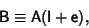(1)

where I is the Identity Matrix. Then the inverse ofis approximately(2)

This can be seen by multiplying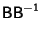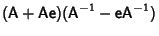(3)

Note that if we instead let, and look for an inverse of the form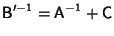, we obtain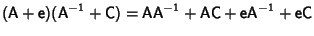(4)

In order to eliminate theterm, we require. However, then, soso there can be no inverse of this form.

The exact inverse ofcan be found as follows.(5)

so(6)

Using a general Matrix Inverse identity then gives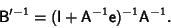(7)